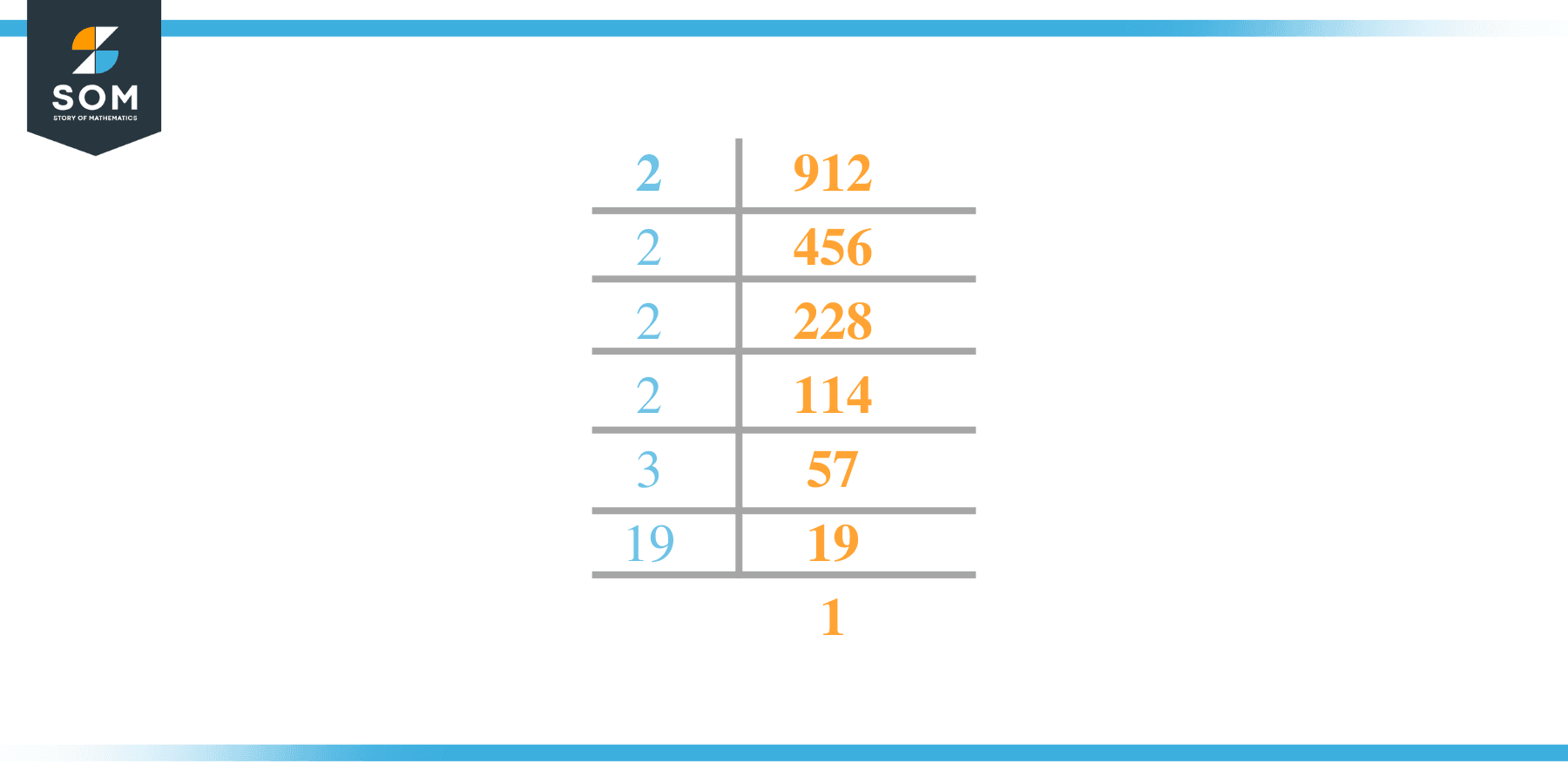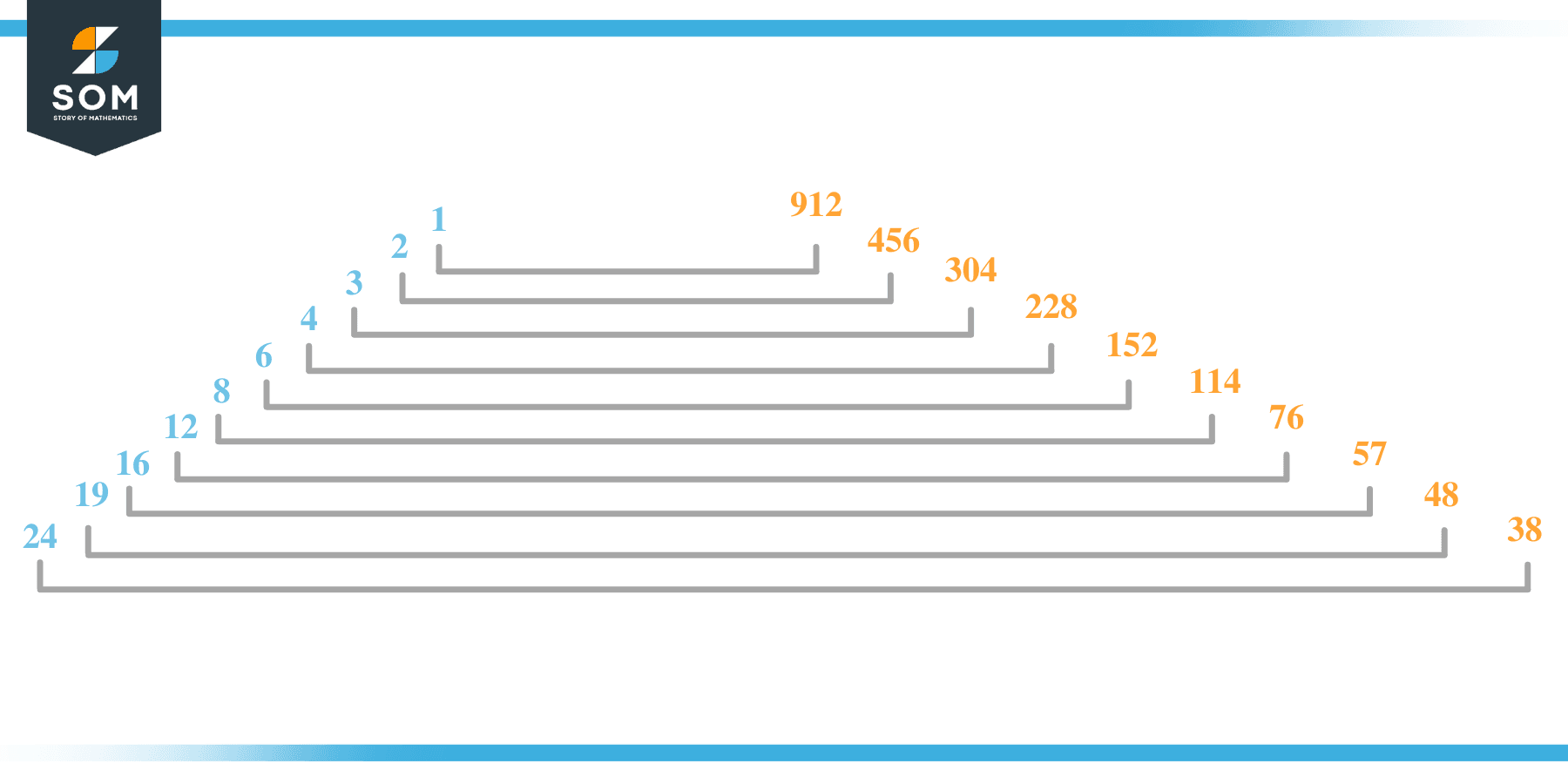# Factors of 912: Prime Factorization, Methods, and Examples

The 912 factors are those numbers that, when divided by 912, result in a remainder of zero. In other words, the factors are the numbers that finally split the supplied number.Given that the given number is obtained by multiplying integers with two factors, its factors may be both positive and negative.

### Factors of 912

Here are the factors of number 912.

Factors of 912: 1, 2, 3, 4, 6, 8, 12, 16, 19, 24, 38, 48, 57, 76, 114, 152, 228, 304, 456 and 912

### Negative Factors of 912

The negative factors of 912 are similar to its positive aspects, just with a negative sign.

Negative Factors of 912: -1, -2, -3,-4, -6, -8, -12, -16, -19, -24, -38, -48, -57, -76, -114, -152, -228, -304, -456 and -912

### Prime Factorization of 912

The prime factorization of 912 is the way of expressing its prime factors in the product form.

Prime Factorization: 24 x 31 x 191

In this article, we will learn about the factors of 912 and how to find them using various techniques such as upside-down division, prime factorization, and factor tree.

## What Are the Factors of 912?

The factors of 912 are 1, 2, 3, 4, 6, 8, 12, 16, 19, 24, 38, 48, 57, 76, 114, 152, 228, 304, 456 and 912. These numbers are the factors as they do not leave any remainder when divided by 912.

The factors of 912 are classified as prime numbers and composite numbers. The prime factors of the number 912 can be determined using the prime factorization technique.

## How To Find the Factors of 912?

You can find the factors of 912 by using the rules of divisibility. The divisibility rule states that any number, when divided by any other natural number, is said to be divisible by the number if the quotient is the whole number and the resulting remainder is zero.

To find the factors of 912, create a list containing the numbers that are exactly divisible by 912 with zero remainders. One important thing to note is that 1 and 912 are the 912’s factors as every natural number has 1 and the number itself as its factor.

1 is also called the universal factor of every number. The factors of 912 are determined as follows:

$\dfrac{912}{1} = 912$

$\dfrac{912}{2} = 456$

$\dfrac{912}{3} = 304$

$\dfrac{912}{4} = 228$

$\dfrac{912}{6} = 152$

$\dfrac{912}{8} = 114$

$\dfrac{912}{12} = 76$

$\dfrac{912}{16} = 57$

$\dfrac{912}{19} = 48$

$\dfrac{912}{24} = 38$

$\dfrac{912}{38} = 24$

$\dfrac{912}{48} = 19$

$\dfrac{912}{57} = 16$

$\dfrac{912}{76} = 12$

$\dfrac{912}{114} = 8$

$\dfrac{912}{152} = 6$

$\dfrac{912}{228} = 4$

$\dfrac{912}{304} = 3$

$\dfrac{912}{456} = 2$

$\dfrac{912}{912} = 1$

Therefore, 1, 2, 3, 4, 6, 8, 12, 16, 19, 24, 38, 48, 57, 76, 114, 152, 228, 304, 456 and 912 are the factors of 912.

### Total Number of Factors of 912

For 912, there are 20 positive factors and 20 negative ones. So in total, there are 40 factors out of 912.

To find the total number of factors of the given number, follow the procedure mentioned below:

1. Find the factorization/prime factorization of the given number.
2. Demonstrate the prime factorization of the number in the form of exponent form.
3. Add 1 to each of the exponents of the prime factor.
4. Now, multiply the resulting exponents together. This obtained product is equivalent to the total number of factors of the given number.

By following this procedure, the total number of factors of 912 is given as:

Factorization of 912 is 24 x 31 x 191.

The exponent of 2 is 4,  3 is 1, and 19 is 1.

Adding 1 to each and multiplying them together results in 40.

Therefore, the total number of factors of 912 is 40. 20 are positive, and 20 factors are negative.

### Important Notes

Here are some essential points that must be considered while finding the factors of any given number:

• The factor of any given number must be a whole number.
• The factors of the number cannot be in the form of decimals or fractions.
• Factors can be positive as well as negative.
• Negative factors are the additive inverse of the positive factors of a given number.
• The factor of a number cannot be greater than that number.
• Every even number has 2 as its prime factor, the smallest prime factor.

## Factors of 912 by Prime FactorizationThe number 912 is a composite number. Prime factorization is a valuable technique for finding the number’s prime factors and expressing the number as the product of its prime factors.

Before finding the factors of 912 using prime factorization, let us find out what prime factors are. Prime factors are the factors of any given number that are only divisible by 1 and themselves.

To start the prime factorization of 912, start dividing by its most minor prime factor. First, determine that the given number is either even or odd. If it is an even number, then 2 will be the smallest prime factor.

Continue splitting the quotient obtained until 1 is received as the quotient. The prime factorization of 912 can be expressed as:

912 =24 x 31 x 191

## Factors of 912 in PairsThe factor pairs are the duplet of numbers that, when multiplied together, result in the factorized number. Factor pairs can be more than one depending on the total number of factors given.

For 912, the factor pairs can be found as:

1 x 912 = 912

2 x 456 = 912

3 x 304 = 912

4 x 228 = 912

6 x 152 = 912

8 x 114 = 912

12 x 76 = 912

16 x 57 = 912

19 x 48 = 912

24 x 38 = 912

The possible factor pairs of 912 are given as (1, 912),(2, 456),(3, 304),(4, 228),(6, 152),(8, 114),(12, 76),(16, 57),(19, 48),and (24, 38).

All these numbers in pairs, when multiplied, give 912 as the product.

The negative factor pairs of 912 are given as:

-1 x -912 = 912

-2 x -456 = 912

-3 x -304 = 912

-4 x -228 = 912

-6 x -152 = 912

-8 x -114 = 912

-12 x -76 = 912

-16 x -57 = 912

-19 x -48 = 912

-24 x -38 = 912

It is important to note that in negative factor pairs, the minus sign has been multiplied by the minus sign, due to which the resulting product is the original positive number. Therefore, -1, -2, -3,-4, -6, -8, -12, -16, -19, -24, -38, -48, -57, -76, -114, -152, -228, -304, -456 and -912 are called negative factors of 912.

The list of all the factors of 912, including positive as well as negative numbers, is given below.

Factor list of 912: 1,-1, 2,-2, 3,-3,4,-4, 6,-6, 8,-8, 12,-12, 16,-16, 19,-19, 24,-24, 38,-38, 48,-48, 57,-57, 76,-76, 114,-114, 152,-152, 228,-228, 304,-304, 456,-456, 912, and -912

## Factors of 912 Solved Examples

To better understand the concept of factors, let’s solve some examples.

### Example 1

How many factors of 912 are there?

### Solution

The total number of Factors of 912 is 40.

Factors of 912 are 1, 2, 3, 4, 6, 8, 12, 16, 19, 24, 38, 48, 57, 76, 114, 152, 228, 304, 456 and 912.

### Example 2

Find the factors of 912 using prime factorization.

### Solution

The prime factorization of 912 is given as:

912 $\div$ 2 = 456

456 $\div$ 2 = 228

228  $\div$ 2 = 114

114  $\div$ 2 = 57

57 $\div$ 3 = 19

19 $\div$ 19 = 1

So the prime factorization of 912 can be written as:

24 x 31 x 191= 912